Chemical Reactions and Writing Chemical Equations Lab Report

Experiment – Studying Chemical Reactions and Writing Chemical

Equations

Directions: To complete the experiment, watch each of the videos below, and use the data provided in the video to complete the experiment.

The experimental report must be submitted to the appropriate Blackboard assignment folder.

Studying Chemical Reactions and Writing Chemical Equations

 Experimental Videos

For each reaction (there are 13 reactions in the video): (Neatness matters) Everything must be typed. All reactants and products must have their physical states indicated. (example H2O (l))

1. Describe the appearance of each reactant.
2. Provide any evidence that a chemical reaction occurred.
3. Write the chemical equation.
4. Write the complete ionic equation.
5. Write the net ionic equation.
6. Name the general reaction type.

A. Neidigeditor:

H.

Studying Chemical Reactions and Writing

Chemical Equations

prepared by M. L. Gillette, Indiana University/Kokomo and H. Anthony Neidig, Lebanon Valley College

 PurpoSe of the ExperimentDescribe chemical reactions by writing chemical equations based on laboratory.observations and information about reactions of different substances.

# Background Information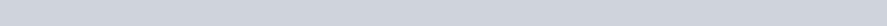The human digestive process depends on a high concentration of hydrochloric acid (HCI) in the stomach. Sometimes the HC} concentration becomes too high, which causes discomfort (“acid stomach’). When this condition occurs, many people take antacids to help neutralize the excess acid. The active ingredient in many antacids is magnesium hydroxide, Mg(OH)2. When solid Mg(OH)2 mixes with HCI solution, a chemical reaction occurs. We can describe this reaction as: Mg(OH)2 reacts with HCI to produce magnesium chloride (MgC12) and water (H20).

We can make the same statement more concisely by writing a chemical equation for the reaction. A chemical equation, the symbolic description of a chemical process, shows the substances that react, the reactants, and the substances that form as a result of a chemical change, the products. An arrow indicates that the process occurs and is read ‘yields.” The chemical equation for the reaction of Mg(OH)2 and HCI is shown in Equation 1.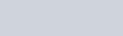+ 2 HCl(aq)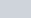MgC12(aq) +2 H20(1) (Eq. 1)The abbreviations in parentheses following each of the chemical formulas indicate the physical state of eachreactant and product. The meanings of the four abbræ viations commonly used in equations are summarized in Table 1.

 Table 1 The meanings of physical-state abbreviations used in chemiæl equations abbreviavon meaning aq substance is dissolved in water substance is a gas at reaction temperature and pressure substance is a pure liquid at reaction temperature and pressure s substance is a solid tiat is in fre reaction mixture47

REAC 422: Studying Chemical Reactions and Writing Chemical Equations

7Ype Ill: Single Displacement Reactions

Tho 2 that appears In front of HCI and 1-120 Is called When one element displaces another element

a coefflclent; It Indicates that two HCI molecules are consumed and two 1-120 molecules are formed In the reaction. Coefficients are used to balance an equation so that tho reaction Involves only a rearrangement of the reactant atoms to form products. When no coefficient is shown, as is the case with Mg(OH)2 and MgC12 in Equation 1, a coefficient of 1 is understood.

The reaction of iron (Fe) with oxygen (02) to form Iron(lll) oxlde (F0203, ferric oxide) is another familiar reaction. The common name for Fe203 Is rust. The chemical equation for this reaction ts given in Equation 2.

## 4 Fe(s) +3 02(g) 2 Fe203(s)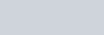Notice an Important difference between Equations I and 2. tn the first reaction, two reactants produce two products whereas In the second reaction, two reactants produce only one product.

Classifying Chemical Reactions

Many chemical reactions are conveniently classified as one of four major types. This classification is based on the type of chemical transformation that occurs.

7Ype l: Combination, Synthesis, or

Formatlon Reactions

As the name suggests, a combination, or syntheSIS reactlon, occurs when two substances combine toform a compound. The reaction In Equation 2 is a combination reaction. Equation 3 is a generalized combination

reaction, while Equations 4—6 are specific examples.A + B * AB8 Mg(s) + 8 Mgs(s) (Eq. 4)

2 ca(s) + 02(g) -i 2 ca0(s)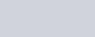H2(g) + C12(g) 2 HCl(g)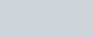7Ype Il: Decomposltlon Reactions

A deeomposltlon reaction occurs when a compound breaks apart to form two or more products. Equation 7 shows a generalized example, while Equations 8—10 give specific examples.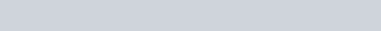(Eq. 7)

2 Hg0(s) 2 Hg(l) + 02(g) (Eq. 8)

from a compound, we call the pFocess a single dis. placement reaction. Equation 11 shows a general, Ized example, and Equations 12 and 13 show specific examples.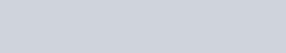(Eq. 11) sn(s) + 2 AgN03(aq)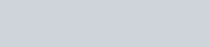+2 Ag(s) (Eq. 12)2 Al(s) + 6 HN03(aq) +3 H2(g) (Eq 13)

2

When we write (aq) after the formula of an ionic compound, we mean that the ions are individuqlty sOIvated by water. We could rewrite Equation 12 as Equation 14 to show the solvation of the ions involved:

Sn(s) + 2 Ag+(aq) + 2 N03¯(aq)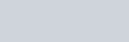+2 NOö(aq) +2 Ag(s) (Eq. 14)

Equation 14 is the complete ionic equation for the reaction. Note that the solvated nitrate ion (NOD appears on both sides of Equation 14. Therefore it is not directly involved in the chemical reaction. We call such ions spectator ions. Thus, Equation 14 may be rewritten with the NOE ion omitted; as In Equation 15.

Sn(s) + 2 Ag+(aq) Sn2+(aq) + 2 Ag(s) (Eq. 15) Equation 15 is the net ionic equation forthis reaction.

Type IV: Double Displacement, DoubleReplacement, or Metathesis ReactionsA double displacement reaction occurs when atoms or ions in two or more different substances change places to form new compounds. One of the new compounds formed Is usually either a solid, called a precipItate, a slightly dissociated compound, such as a gas. A doubte displacement reaction involving the formation of a precipitate is often called a precipitation reaction. Equation 16 is a general example; Equation 17 is a specific example.(Eq. 16)

The complete and net ionic equations NaN03(aq) for the reaction (Eq. 17)in

Fquatlon 17 are shown in Equations 18 and 19,

 BaC03(s) Ba0(s) + C02(g) (Eq. 9)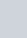Pb2+(aq) + 2 NOö(aq) + 2 Na+(aq) + 2 ct-(aq) -+ 2 Pb02(s) -9 2 Pb0(s) + 02(g) (Eq. 10)PbC12(s) +2 2 NOö(aa) (Ea. 18)

C 422: Studying Chemical Reactions and Writing Chemical Equations

 If you spill any reagent on your hands or skin, immediately wash with ample amounts of running water.

Pb2+(aq) + 2 cr(aq) -4 PbC12(s) (Eq. 19)

 Note: The numbers appearing in parentheses indicate the specific lines on your Data Sheet onwhich the indicated data should be entered.

In this experiment, you will mix different elements and/or components in solution. In some cases, you will heat th’e mixtures to promote a reaction. You will observe the appearance of the reactants and of the products, and you will determine which type of reaction occurs in each case. You will also write the chemical equations for the reactions you perform. The chemical symbols for the elements and compounds you will work with are listed in Table 2. I. Reacting Mg with HCI Solution

 Table 2 Chemical symbols for the elements and compounds used in this experiment name chemical symbol ammonia NH3 ammonium carbonate (NH4)2C03 carbon(lV) oxide (carbon dioxide) C02copper cu copper(ll) sulfate (cupric sulfate) cuS04 copper(ll) sulfate pentahydrate CuSOC 5 H20 • hydrochloric acid iron iron(lll) chloride (ferric chloride) lead(ll) nitrate magnesium Mg oxygen 02 potassium iodidesodium hydrpxide NaOHsulfur
 Caution: The gas produced by the reaction of Mg and HCI solution is flammable. Be sure that there are no Bunsen burner flames in the area where you are performing this reaction.
1.Transfera 0.5-cm piece of Mg ribbon to the bottom of a clean test tube.

Record on your Data Sheet your description of the appearance of the Mg (1).

1.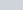Measure 2 mL of 0.1M HCI solution in a 10-mL graduated cylinder. Record on your Data Sheet your description of the appearance ofthe HCI solution (2).

Transferthe HCI solution to the test tube containing the Mg. Observe the reaction mixture for evidence of a chemical reaction.

Record on your Data Sheet any evidence that a chemical reaction has occurred (3).

1. Transfer the contents of your test tube to the container specified by your laboratory instructor and labe!ed “Discarded Mg—HCI Reaction Mixtures.”

# Procedure

 Note: Your laboratory instructor will .tell you whether or not you should complete Step 4 before proceeding to Step 5.
 Chemical Alertammonia—toxic and corrosive ammonium carbonate—irritant0.1 M copper(ll) sulfate—toxic and IrritantO. 1 M hydrochloric acid—toxic and corrosiveO. 1 M iron(lll) chloride—corrosive0.1 M lead(ll) nitrate—toxic, irritant, and oxidantmagnesium—flammable0.1 M potassium iodide—toxic and irritant0.1 M sodium hydroxide—toxic and corrosive sulfur—irritant Caution: Wear departmentally approved eye protection while doing this experiment.
 Caution: Lead nitrate and KI solutions are toxic. Immediately notify your laboratory instructor if any solution spills.

4. Write on your Data Sheet the complete and net ionic equations for the reaction of Mg with HCI solution (4, 5). Indicate which of the four general reaction types is represented by this reaction (6).Il. Reacting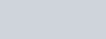Solution with Kl

Solution

49

A. Neidigeditor:

H.

Studying Chemical Reactions and Writing

Chemical Equations

prepared by M. L. Gillette, Indiana University/Kokomo and H. Anthony Neidig, Lebanon Valley College

 PurpoSe of the ExperimentDescribe chemical reactions by writing chemical equations based on laboratory.observations and information about reactions of different substances.

# Background InformationThe human digestive process depends on a high concentration of hydrochloric acid (HCI) in the stomach. Sometimes the HC} concentration becomes too high, which causes discomfort (“acid stomach’). When this condition occurs, many people take antacids to help neutralize the excess acid. The active ingredient in many antacids is magnesium hydroxide, Mg(OH)2. When solid Mg(OH)2 mixes with HCI solution, a chemical reaction occurs. We can describe this reaction as: Mg(OH)2 reacts with HCI to produce magnesium chloride (MgC12) and water (H20).

We can make the same statement more concisely by writing a chemical equation for the reaction. A chemical equation, the symbolic description of a chemical process, shows the substances that react, the reactants, and the substances that form as a result of a chemical change, the products. An arrow indicates that the process occurs and is read ‘yields.” The chemical equation for the reaction of Mg(OH)2 and HCI is shown in Equation 1.+ 2 HCl(aq)MgC12(aq) +2 H20(1) (Eq. 1)The abbreviations in parentheses following each of the chemical formulas indicate the physical state of eachreactant and product. The meanings of the four abbræ viations commonly used in equations are summarized in Table 1.

 Table 1 The meanings of physical-state abbreviations used in chemiæl equations abbreviavon meaning aq substance is dissolved in water substance is a gas at reaction temperature and pressure substance is a pure liquid at reaction temperature and pressure s substance is a solid tiat is in fre reaction mixture47

REAC 422: Studying Chemical Reactions and Writing Chemical Equations

7Ype Ill: Single Displacement Reactions

Tho 2 that appears In front of HCI and 1-120 Is called When one element displaces another element

a coefflclent; It Indicates that two HCI molecules are consumed and two 1-120 molecules are formed In the reaction. Coefficients are used to balance an equation so that tho reaction Involves only a rearrangement of the reactant atoms to form products. When no coefficient is shown, as is the case with Mg(OH)2 and MgC12 in Equation 1, a coefficient of 1 is understood.

The reaction of iron (Fe) with oxygen (02) to form Iron(lll) oxlde (F0203, ferric oxide) is another familiar reaction. The common name for Fe203 Is rust. The chemical equation for this reaction ts given in Equation 2.

## 4 Fe(s) +3 02(g) 2 Fe203(s)Notice an Important difference between Equations I and 2. tn the first reaction, two reactants produce two products whereas In the second reaction, two reactants produce only one product.

Classifying Chemical Reactions

Many chemical reactions are conveniently classified as one of four major types. This classification is based on the type of chemical transformation that occurs.

7Ype l: Combination, Synthesis, or

Formatlon Reactions

As the name suggests, a combination, or syntheSIS reactlon, occurs when two substances combine toform a compound. The reaction In Equation 2 is a combination reaction. Equation 3 is a generalized combination

reaction, while Equations 4—6 are specific examples.A + B * AB8 Mg(s) + 8 Mgs(s) (Eq. 4)

2 ca(s) + 02(g) -i 2 ca0(s)H2(g) + C12(g) 2 HCl(g)7Ype Il: Decomposltlon Reactions

A deeomposltlon reaction occurs when a compound breaks apart to form two or more products. Equation 7 shows a generalized example, while Equations 8—10 give specific examples.(Eq. 7)

2 Hg0(s) 2 Hg(l) + 02(g) (Eq. 8)

from a compound, we call the pFocess a single dis. placement reaction. Equation 11 shows a general, Ized example, and Equations 12 and 13 show specific examples.(Eq. 11) sn(s) + 2 AgN03(aq)+2 Ag(s) (Eq. 12)2 Al(s) + 6 HN03(aq) +3 H2(g) (Eq 13)

2

When we write (aq) after the formula of an ionic compound, we mean that the ions are individuqlty sOIvated by water. We could rewrite Equation 12 as Equation 14 to show the solvation of the ions involved:

Sn(s) + 2 Ag+(aq) + 2 N03¯(aq)+2 NOö(aq) +2 Ag(s) (Eq. 14)

Equation 14 is the complete ionic equation for the reaction. Note that the solvated nitrate ion (NOD appears on both sides of Equation 14. Therefore it is not directly involved in the chemical reaction. We call such ions spectator ions. Thus, Equation 14 may be rewritten with the NOE ion omitted; as In Equation 15.

Sn(s) + 2 Ag+(aq) Sn2+(aq) + 2 Ag(s) (Eq. 15) Equation 15 is the net ionic equation forthis reaction.

Type IV: Double Displacement, DoubleReplacement, or Metathesis ReactionsA double displacement reaction occurs when atoms or ions in two or more different substances change places to form new compounds. One of the new compounds formed Is usually either a solid, called a precipItate, a slightly dissociated compound, such as a gas. A doubte displacement reaction involving the formation of a precipitate is often called a precipitation reaction. Equation 16 is a general example; Equation 17 is a specific example.(Eq. 16)

The complete and net ionic equations NaN03(aq) for the reaction (Eq. 17)in

Fquatlon 17 are shown in Equations 18 and 19,

 BaC03(s) Ba0(s) + C02(g) (Eq. 9)Pb2+(aq) + 2 NOö(aq) + 2 Na+(aq) + 2 ct-(aq) -+ 2 Pb02(s) -9 2 Pb0(s) + 02(g) (Eq. 10)PbC12(s) +2 2 NOö(aa) (Ea. 18)

C 422: Studying Chemical Reactions and Writing Chemical Equations

 If you spill any reagent on your hands or skin, immediately wash with ample amounts of running water.

Pb2+(aq) + 2 cr(aq) -4 PbC12(s) (Eq. 19)

 Note: The numbers appearing in parentheses indicate the specific lines on your Data Sheet onwhich the indicated data should be entered.

In this experiment, you will mix different elements and/or components in solution. In some cases, you will heat th’e mixtures to promote a reaction. You will observe the appearance of the reactants and of the products, and you will determine which type of reaction occurs in each case. You will also write the chemical equations for the reactions you perform. The chemical symbols for the elements and compounds you will work with are listed in Table 2. I. Reacting Mg with HCI Solution

 Table 2 Chemical symbols for the elements and compounds used in this experiment name chemical symbol ammonia NH3 ammonium carbonate (NH4)2C03 carbon(lV) oxide (carbon dioxide) C02copper cu copper(ll) sulfate (cupric sulfate) cuS04 copper(ll) sulfate pentahydrate CuSOC 5 H20 • hydrochloric acid iron iron(lll) chloride (ferric chloride) lead(ll) nitrate magnesium Mg oxygen 02 potassium iodide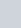sodium hydrpxide NaOHsulfur
 Caution: The gas produced by the reaction of Mg and HCI solution is flammable. Be sure that there are no Bunsen burner flames in the area where you are performing this reaction.
1.Transfera 0.5-cm piece of Mg ribbon to the bottom of a clean test tube.

Record on your Data Sheet your description of the appearance of the Mg (1).

1.Measure 2 mL of 0.1M HCI solution in a 10-mL graduated cylinder. Record on your Data Sheet your description of the appearance ofthe HCI solution (2).

Transferthe HCI solution to the test tube containing the Mg. Observe the reaction mixture for evidence of a chemical reaction.

Record on your Data Sheet any evidence that a chemical reaction has occurred (3).

1. Transfer the contents of your test tube to the container specified by your laboratory instructor and labe!ed “Discarded Mg—HCI Reaction Mixtures.”

# Procedure

 Note: Your laboratory instructor will .tell you whether or not you should complete Step 4 before proceeding to Step 5.
 Chemical Alertammonia—toxic and corrosive ammonium carbonate—irritant0.1 M copper(ll) sulfate—toxic and IrritantO. 1 M hydrochloric acid—toxic and corrosiveO. 1 M iron(lll) chloride—corrosive0.1 M lead(ll) nitrate—toxic, irritant, and oxidantmagnesium—flammable0.1 M potassium iodide—toxic and irritant0.1 M sodium hydroxide—toxic and corrosive sulfur—irritant Caution: Wear departmentally approved eye protection while doing this experiment.
 Caution: Lead nitrate and KI solutions are toxic. Immediately notify your laboratory instructor if any solution spills.

4. Write on your Data Sheet the complete and net ionic equations for the reaction of Mg with HCI solution (4, 5). Indicate which of the four general reaction types is represented by this reaction (6).Il. ReactingSolution with Kl

Solution

49